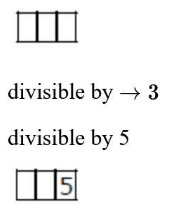# The total number of numbers,Question:

The total number of numbers, lying between 100 and 1000 that can be formed with the digits $1,2,3,4,5$, if the repetition of digits is not allowed and numbers are divisible by either 3 or 5 is

Solution:$=12$

$4 \times 3$

$12 \rightarrow 3,4,5 \rightarrow 3 !=6$

$15 \rightarrow 2,3,4 \rightarrow 3 !=6$

$24 \rightarrow 1,3,5 \rightarrow 3 !=6$

$42 \rightarrow 1,2,3 \rightarrow 3 !=6$

Required No. $=24+12-4=32$